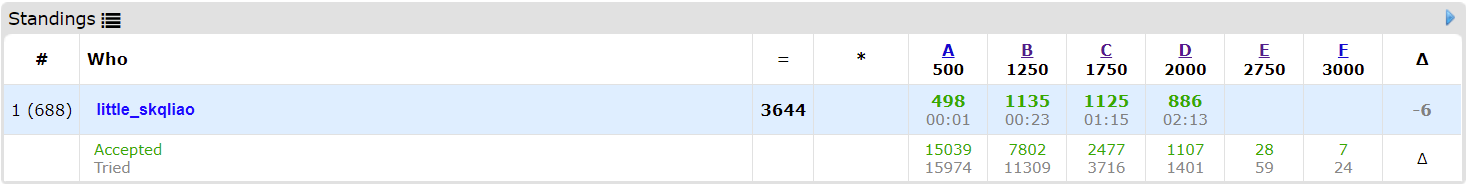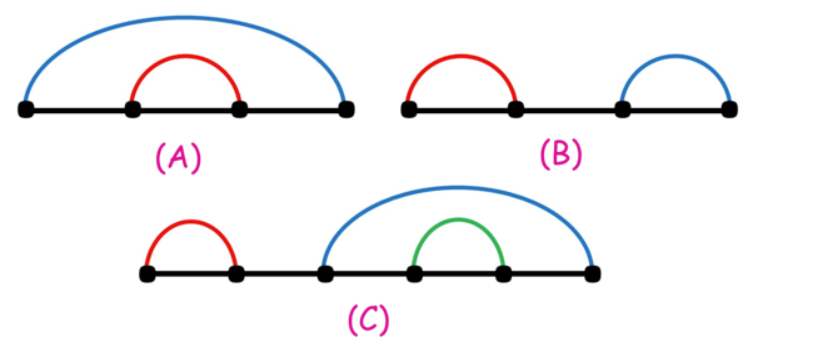rank600+为啥还会掉，之前rank800+不还涨了吗，哭哭。。

long long！！！Code

Code

## C - Parsa’s Humongous Tree

$n\leq 2\times 10^5$，$1\leq l_i,r_i\leq 10^9$

$f_{u,0/1}$ 表示当点 $a_u$ 的权值为 $l_u/r_u$ 时，以 $u$ 为根的子树的边权之和的最大值。

$f_{u,0}=\sum\limits_{v\in son(u)}{\max(f_{v,0}+|l_u-l_v|,f_{v,1}+|l_u-r_v|)}$

$f_{u,1}=\sum\limits_{v\in son(u)}{\max(f_{v,0}+|r_u-l_v|,f_{v,1}+|r_u-r_v|)}$

Code

## D - Kavi on Pairing Duty

X轴上有 $2n$ 个点，第 $i$ 个点的坐标为 $i$。

• $r_i-l_i=r_j-l_j$
• $l_i<l_j<r_j<r_i$ 或 $l_j<l_i<r_i<r_j$$n\leq 10^6$

Code

## E - Trees of Tranquillity

$n\leq 3\times 10^5$

• 插入点 $x$
• 删除点 $x$
• 查询集合中点的个数

DFS回溯到 $x$时，我们要撤销 $x$ 的染色，并将 $l[x]$ （若存在）的子树染色。

Code